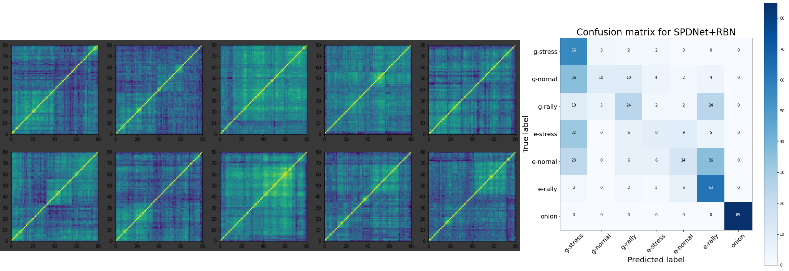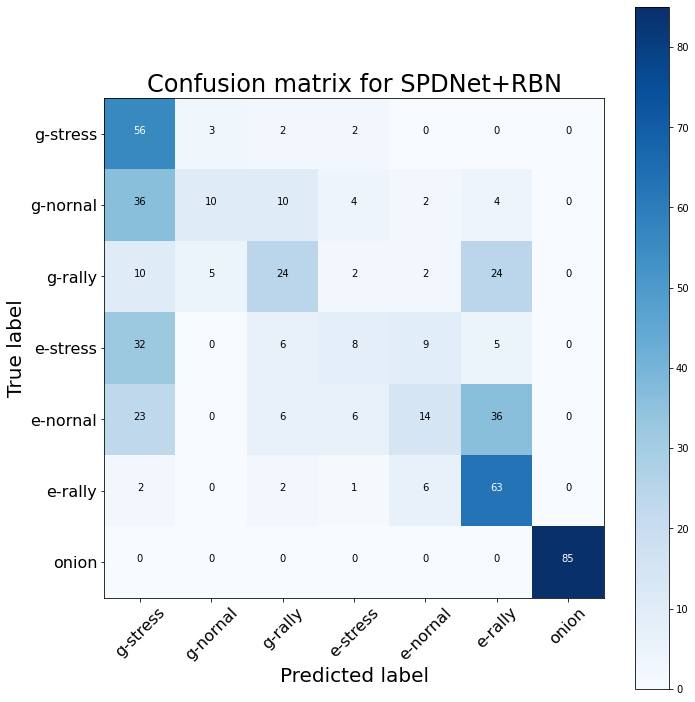A few cCorrGAN-generated correlation matrices, and the confusion matrix of a SPDNet + RBN classification.

# Conditional CorrGAN: An example in Google Colab

Victor Goubet, Chloé Daems, Thomas Graff, Quentin Bourgue, students from the engineering school ESILV, have worked toward extending the original CorrGAN model to a conditional variant: Sampling random realistic financial correlation matrices conditional on a market regime: stressed (stocks are plummeting), normal (business as usual), rally (stocks prices skyrocketing).

In this Google Colab, you can have a look at how to use a trained model.

Note that the model is still in development and far from perfect. Work in progress…

Using SPDNet with Riemannian Batch Normalization (a different architecture than the one used in the discriminator part of the conditional CorrGAN model) to classify a dataset of correlation matrices into one of the seven following classes:

1. g-stress (cCorrGAN generated stressed correlation matrix)
2. g-normal (cCorrGAN generated normal correlation matrix)
3. g-rally (cCorrGAN generated rally correlation matrix)
4. e-stress (empirical stressed correlation matrix)
5. e-normal (empirical normal correlation matrix)
6. e-rally (empirical rally correlation matrix)
7. onion (correlation matrix generated by the onion method)

we obtain the following confusion matrix:A confusion matrix of the classification obtained using SPDNet with Riemannian Batch Normalization: This particular instance of the Conditional CorrGAN model is good at generating realistic ‘rally’ correlation matrices (the classifier cannot easily distinguish between the empirical ones and the GAN-generated ones). Less so for the other two regimes.